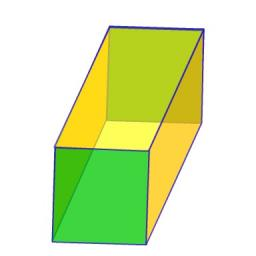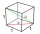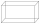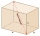# Prism

The lenght, width and height of a right prism are 17, 11 and 11 respectively. What is the lenght of the longest segment whose endpoints are vertices of the prism?

Correct result:

d =  23.0434

#### Solution:We would be pleased if you find an error in the word problem, spelling mistakes, or inaccuracies and send it to us. Thank you!Tips to related online calculators
Do you want to convert length units?
Pythagorean theorem is the base for the right triangle calculator.

#### You need to know the following knowledge to solve this word math problem:

We encourage you to watch this tutorial video on this math problem:

## Next similar math problems:

• Concrete blockDetermine the volume of concrete block whose one edge of the base has a length 3 meters, body diagonal is 13 meters and its height is 12 meters.
• Find diagonalFind diagonal of cuboid with length=20m width=25m height=150m
• Cuboidal roomLength of cuboidal room is 2m breadth of cuboidal room is 3m and height is 6m find the length of the longest rod that can be fitted in the room
• Four sided prismCalculate the volume and surface area of a regular quadrangular prism whose height is 28.6cm and the body diagonal forms a 50 degree angle with the base plane.
• Regular hexagonal prismCalculate the volume of a regular hexagonal prism whose body diagonals are 24cm and 25cm long.
• Rhombus baseCalculate the volume and surface area of prisms whose base is a rhombus with diagonals u1 = 12 cm and u2 = 15 cm. Prism height is twice base edge length.
• Height of the roomGiven the floor area of a room as 24 feet by 48 feet and space diagonal of a room as 56 feet. Can you find the height of the room?The height of a regular quadrilateral prism is v = 10 cm, the deviation of the body diagonal from the base is 60°. Determine the length of the base edges, the surface, and the volume of the prism.
• A boxA box is 15 centimeters long, 4 centimeters wide, and 3 centimeters tall what is the diagonal S of the bottom side? What is the length of the body diagnol R?
• Body diagonalCalculate the volume of a cuboid whose body diagonal u is equal to 6.1 cm. Rectangular base has dimensions of 3.2 cm and 2.4 cmCalculate the volume (V) and the surface (S) of a regular quadrilateral prism whose height is 28.6 cm and the deviation of the body diagonal from the base plane is 50°.Calculate the cube volume, whose body diagonal size is 75 dm. Draw a picture and highlight the body diagonal.The dimensions of the cuboid are in a ratio 3: 1: 2. The body diagonal has a length of 28 cm. Find the volume of a cuboid.Determine the dimensions of the cuboid, if diagonal long 53 dm has angle with one edge 42° and with other edge 64°.The block/cuboid has dimensions a = 4cm, b = 3cm and c = 12cm. Calculates the length of the wall and body diagonals.Regular tetrahedral pyramid has a base edge a=17 and collaterally edge length b=32. What is its height?The space diagonal of a cube is 129.91 mm. Find the lateral area, surface area and the volume of the cube.